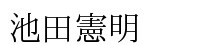Noriaki Ikeda[English/Japanese]

Department of Mathematical Sciences, Ritsumeikan University,
Kusatsu, 525-8577, Japan

Research Interests:

Gauge Theory and Topological Field Theory, Poisson Geometry and Supergeometry, Mathematical Physics

• Mathematical structures in physical theories, especially, gauge theories. Analysis of mathematical theories from physical ideas.
• Keywords: Poisson sigma models, Courant sigma models, AKSZ sigma models, Groupoids and algebroids, Supermanifolds, T-duality.